Need help? Email help@shout.education

# Lattice Enthalpy (Lattice Energy)

Lattice enthalpy and lattice energy are commonly used as if they mean exactly the same thing – you will often find both terms used within the same textbook article or web site, including on university sites.

In fact, there is a difference between them which relates to the conditions under which they are calculated. However, the difference is small, and negligible compared with the differing values for lattice enthalpy that you will find from different data sources.

Unless you go on to do chemistry at degree level, the difference between the two terms isn't likely to worry you.

Note: While I have been writing this section, the different values for the same piece of data from different data sources has driven me crazy, because there is no easy way of knowing which is the most recent or most accurate data.

In the Born-Haber cycles below, I have used numbers which give a consistent answer, but please don't assume that they are necessarily the most accurate ones. If you are doing a course for 16 – 18 year olds, none of this really matters – you just use the numbers you are given.

## What is Lattice Enthalpy?

### Two Different Ways of Defining Lattice Enthalpy

There are two different ways of defining lattice enthalpy which directly contradict each other, and you will find both in common use. In fact, there is a simple way of sorting this out, but many sources don't use it.

I will explain how you can do this in a moment, but first let's look at how the problem arises.

Lattice enthalpy is a measure of the strength of the forces between the ions in an ionic solid. The greater the lattice enthalpy, the stronger the forces.

Those forces are only completely broken when the ions are present as gaseous ions, scattered so far apart that there is negligible attraction between them. You can show this on a simple enthalpy diagram.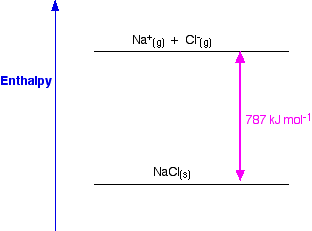For sodium chloride, the solid is more stable than the gaseous ions by 787 kJ mol-1, and that is a measure of the strength of the attractions between the ions in the solid. Remember that energy (in this case heat energy) is given out when bonds are made, and is needed to break bonds.

So lattice enthalpy could be described in either of two ways.

• You could describe it as the enthalpy change when 1 mole of sodium chloride (or whatever) was formed from its scattered gaseous ions. In other words, you are looking at a downward arrow on the diagram.
In the sodium chloride case, that would be -787 kJ mol-1.
• Or, you could describe it as the enthalpy change when 1 mole of sodium chloride (or whatever) is broken up to form its scattered gaseous ions.In other words, you are looking at an upward arrow on the diagram.
In the sodium chloride case, that would be +787 kJ mol-1.

Both refer to the same enthalpy diagram, but one looks at it from the point of view of making the lattice, and the other from the point of view of breaking it up.

Unfortunately, both of these are often described as "lattice enthalpy".

This is an absurdly confusing situation which is easily resolved. I suggest that you never use the term "lattice enthalpy" without qualifying it.

• You should talk about "lattice dissociation enthalpy" if you want to talk about the amount of energy needed to split up a lattice into its scattered gaseous ions.
For NaCl, the lattice dissociation enthalpy is +787 kJ mol-1.
• You should talk about "lattice formation enthalpy" if you want to talk about the amount of energy released when a lattice is formed from its scattered gaseous ions.
For NaCl, the lattice formation enthalpy is -787 kJ mol-1.

That immediately removes any possibility of confusion.

##### So

Lattice dissociation enthalpy: the enthalpy change needed to convert 1 mole of solid crystal into its scattered gaseous ions. Lattice dissociation enthalpies are always positive.

Lattice formation enthalpy: the enthalpy change when 1 mole of solid crystal is formed from its scattered gaseous ions. Lattice formation enthalpies are always negative.

Note: Find out which of these versions your syllabus is likely to want you to know (even if they just call it "lattice enthalpy") and concentrate on that one, but be aware of the confusion!

Incidentally, if you are ever uncertain about which version is being used, you can tell from the sign of the enthalpy change being discussed. If the sign is positive, for example, it must refer to breaking bonds, and therefore to a lattice dissociation enthalpy.

## Factors affecting lattice enthalpy

The two main factors affecting lattice enthalpy are the charges on the ions and the ionic radii (which affects the distance between the ions).

### The Charges on the Ions

Sodium chloride and magnesium oxide have exactly the same arrangements of ions in the crystal lattice, but the lattice enthalpies are very different.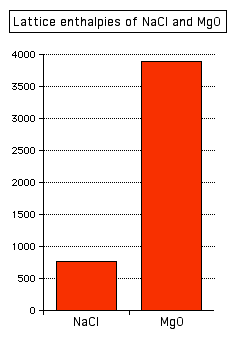Note: In this diagram, and similar diagrams below, I am not interested in whether the lattice enthalpy is defined as a positive or a negative number – I am just interested in their relative sizes. Strictly speaking, because I haven't added a sign to the vertical axis, the values are for lattice dissociation enthalpies. If you prefer lattice formation enthalpies, just mentally put a negative sign in front of each number.

You can see that the lattice enthalpy of magnesium oxide is much greater than that of sodium chloride. That's because in magnesium oxide, 2+ ions are attracting 2- ions; in sodium chloride, the attraction is only between 1+ and 1- ions.

### The Radius of the Ions

The lattice enthalpy of magnesium oxide is also increased relative to sodium chloride because magnesium ions are smaller than sodium ions, and oxide ions are smaller than chloride ions.

That means that the ions are closer together in the lattice, and that increases the strength of the attractions.

You can also see this effect of ion size on lattice enthalpy as you go down a Group in the Periodic Table.

For example, as you go down Group 7 of the Periodic Table from fluorine to iodine, you would expect the lattice enthalpies of their sodium salts to fall as the negative ions get bigger – and that is the case: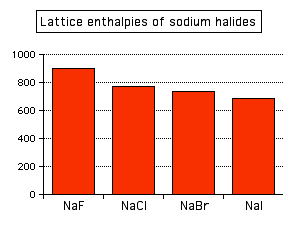Attractions are governed by the distances between the centres of the oppositely charged ions, and that distance is obviously greater as the negative ion gets bigger.

And you can see exactly the same effect as you go down Group 1. The next bar chart shows the lattice enthalpies of the Group 1 chlorides.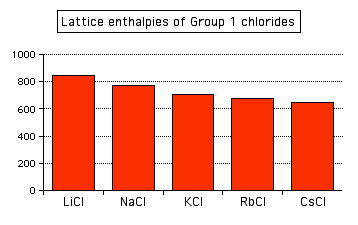Note: To save anyone the bother of getting in touch with me to point it out, it's not strictly fair to include caesium chloride in this list. Caesium chloride has a different packing arrangement of ions in its crystal, and that has a small effect on the lattice enthalpy. The effect is small enough that it doesn't actually affect the trend.

## Calculating Lattice Enthalpy

It is impossible to measure the enthalpy change starting from a solid crystal and converting it into its scattered gaseous ions. It is even more difficult to imagine how you could do the reverse – start with scattered gaseous ions and measure the enthalpy change when these convert to a solid crystal.

Instead, lattice enthalpies always have to be calculated, and there are two entirely different ways in which this can be done.

You can can use a Hess's Law cycle (in this case called a Born-Haber cycle) involving enthalpy changes which can be measured. Lattice enthalpies calculated in this way are described as experimental values.

Or you can do physics-style calculations working out how much energy would be released, for example, when ions considered as point charges come together to make a lattice. These are described as theoretical values. In fact, in this case, what you are actually calculating are properly described as lattice energies.

Note: If you aren't confident about Hess's Law cycles, it is essential that you follow this link before you go on.

### Experimental Values – Born-Haber Cycles

#### Standard atomisation enthalpies

Before we start talking about Born-Haber cycles, there is an extra term which we need to define. That is atomisation enthalpy, ΔH°a.

Standard atomisation enthalpy: the enthalpy change when 1 mole of gaseous atoms is formed from the element in its standard state. Enthalpy change of atomisation is always positive.

You are always going to have to supply energy to break an element into its separate gaseous atoms.

All of the following equations represent changes involving atomisation enthalpy:

\begin{matrix} \frac{1}{2}\text{Cl}_{2(g)} \longrightarrow \text{Cl}_{(g)} & \Delta H^o_a = {+}122 \text{ kJ mol}^{-1} \\ \\ \frac{1}{2}\text{Br}_{2(g)} \longrightarrow \text{Br}_{(g)} & \Delta H^o_a = {+}112 \text{ kJ mol}^{-1} \\ \\ \text{Na}_{(s)} \longrightarrow \text{Na}_{(g)} & \Delta H^o_a = {+}107 \text{ kJ mol}^{-1} \end{matrix}

Notice particularly that the "mol-1" is per mole of atoms formed – NOT per mole of element that you start with. You will quite commonly have to write fractions into the left-hand side of the equation. Getting this wrong is a common mistake.

#### Born-Haber cycles

I am going to start by drawing a Born-Haber cycle for sodium chloride, and then talk it through carefully afterwards. You will see that I have arbitrarily decided to draw this for lattice formation enthalpy. If you wanted to draw it for lattice dissociation enthalpy, the red arrow would be reversed – pointing upwards.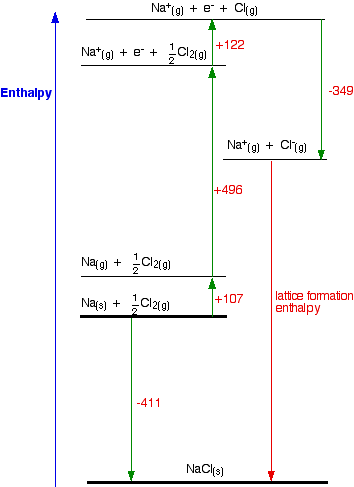Focus to start with on the higher of the two thicker horizontal lines. We are starting here with the elements sodium and chlorine in their standard states. Notice that we only need half a mole of chlorine gas in order to end up with 1 mole of NaCl.

The arrow pointing down from this to the lower thick line represents the enthalpy change of formation of sodium chloride.

The Born-Haber cycle now imagines this formation of sodium chloride as happening in a whole set of small changes, most of which we know the enthalpy changes for – except, of course, for the lattice enthalpy that we want to calculate.

• The +107 is the atomisation enthalpy of sodium. We have to produce gaseous atoms so that we can use the next stage in the cycle.
• The +496 is the first ionisation energy of sodium. Remember that first ionisation energies go from gaseous atoms to gaseous singly charged positive ions.
• The +122 is the atomisation enthalpy of chlorine. Again, we have to produce gaseous atoms so that we can use the next stage in the cycle.
• The -349 is the first electron affinity of chlorine. Remember that first electron affinities go from gaseous atoms to gaseous singly charged negative ions.
• And finally, we have the positive and negative gaseous ions that we can convert into the solid sodium chloride using the lattice formation enthalpy.

Note: If you have forgotten about ionisation energies or electron affinities follow these links before you go on.

Now we can use Hess's Law and find two different routes around the diagram which we can equate.

As I have drawn it, the two routes are obvious. The diagram is set up to provide two different routes between the thick lines.

So, here is the cycle again, with the calculation directly underneath it\begin{aligned} {-}411 &= 107 + 496 + 122 - 349 + LE \\ LE &= {-}411 - 107 - 496 - 122 + 349 \\ LE &= {-}787 \text{ kJ mol}^{-1} \end{aligned}

Note: Notice that in the calculation, we aren't making any assumptions about the sign of the lattice enthalpy (despite the fact that it is obviously negative because the arrow is pointing downwards). In the first line of the calculation, I have just written "+ LE", and have left it to the calculation to work out that it is a negative answer.

How would this be different if you had drawn a lattice dissociation enthalpy in your diagram? (Perhaps because that is what your syllabus wants.)

Your diagram would now look like this: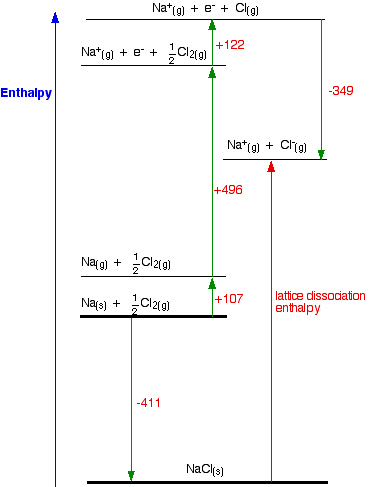The only difference in the diagram is the direction the lattice enthalpy arrow is pointing. It does, of course, mean that you have to find two new routes. You can't use the original one, because that would go against the flow of the lattice enthalpy arrow.

This time both routes would start from the elements in their standard states, and finish at the gaseous ions.

\begin{aligned} {-}411 + LE &= 107 + 496 + 122 - 349 \\ LE &= 107 + 496 + 122 - 349 + 411 \\ LE &= {+}787 \text{ kJ mol}^{-1} \end{aligned}

Once again, the cycle sorts out the sign of the lattice enthalpy for you.

Note: You will find more examples of calculations involving Born-Haber cycles in my chemistry calculations book. This includes rather more complicated cycles involving, for example, oxides.

If you compare the figures in the book with the figures for NaCl above, you will find slight differences – the main culprit being the electron affinity of chlorine, although there are other small differences as well. Don't worry about this – the values in the book come from an older data source. In an exam, you will just use the values you are given, so it isn't a problem.

### Theoretical Values for Lattice Energy

Let's assume that a compound is fully ionic. Let's also assume that the ions are point charges – in other words that the charge is concentrated at the centre of the ion. By doing physics-style calculations, it is possible to calculate a theoretical value for what you would expect the lattice energy to be.

And no – I am not being careless about this! Calculations of this sort end up with values of lattice energy, and not lattice enthalpy. If you know how to do it, you can then fairly easily convert between the two.

There are several different equations, of various degrees of complication, for calculating lattice energy in this way. You won't be expected to be able to do these calculations at this level, but you might be expected to comment on the results of them.

There are two possibilities:

• There is reasonable agreement between the experimental value (calculated from a Born-Haber cycle) and the theoretical value.
Sodium chloride is a case like this – the theoretical and experimental values agree to within a few percent. That means that for sodium chloride, the assumptions about the solid being ionic are fairly good.
• The experimental and theoretical values don't agree.
A commonly quoted example of this is silver chloride, AgCl. Depending on where you get your data from, the theoretical value for lattice enthalpy for AgCl is anywhere from about 50 to 150 kJ mol-1 less than the value that comes from a Born-Haber cycle.
In other words, treating the AgCl as 100% ionic underestimates its lattice enthalpy by quite a lot.
The explanation is that silver chloride actually has a significant amount of covalent bonding between the silver and the chlorine, because there isn't enough electronegativity difference between the two to allow for complete transfer of an electron from the silver to the chlorine.

Comparing experimental (Born-Haber cycle) and theoretical values for lattice enthalpy is a good way of judging how purely ionic a crystal is.

Note: If you have forgotten about electronegativity it might pay you to revise it now by following this link.

## Why is magnesium chloride MgCl2?

This section may well go beyond what your syllabus requires. Before you spend time on it, check your syllabus (and past exam papers as well if possible) to make sure.

The question arises as to why, from an energetics point of view, magnesium chloride is MgCl2 rather than MgCl or MgCl3 (or any other formula you might like to choose).

It turns out that MgCl2 is the formula of the compound which has the most negative enthalpy change of formation – in other words, it is the most stable one relative to the elements magnesium and chlorine.

##### Let's look at this in terms of Born-Haber cycles.

In the cycles this time, we are interested in working out what the enthalpy change of formation would be for the imaginary compounds MgCl and MgCl3.

That means that we will have to use theoretical values of their lattice enthalpies. We can't use experimental ones, because these compounds obviously don't exist!

I'm taking theoretical values for lattice enthalpies for these compounds that I found on the web. I can't confirm these, but all the other values used by that source were accurate. The exact values don't matter too much anyway, because the results are so dramatically clear-cut.

We will start with the compound MgCl, because that cycle is just like the NaCl one we have already looked at.

### The Born-Haber Cycle for MgCl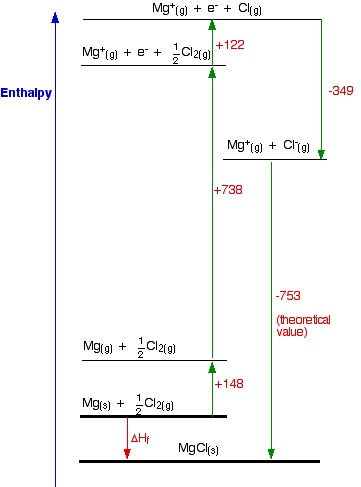Find two routes around this without going against the flow of any arrows. That's easy:

\begin{aligned} \Delta H_f &= 148 + 738 + 122 - 349 - 753 \\ \Delta H_f &= {-}94 \text{ kJ mol}^{-1} \end{aligned}

So the compound MgCl is definitely energetically more stable than its elements.

I have drawn this cycle very roughly to scale, but that is going to become more and more difficult as we look at the other two possible formulae. So I am going to rewrite it as a table.

You can see from the diagram that the enthalpy change of formation can be found just by adding up all the other numbers in the cycle, and we can do this just as well in a table.

kJ
atomisation enthalpy of Mg+148
1st IE of Mg+738
atomisation enthalpy of Cl+122
electron affinity of Cl-349
lattice enthalpy-753
calculated ΔHf-94

### The Born-Haber cycle for MgCl2

The equation for the enthalpy change of formation this time is

\text{Mg}_{(s)} + \text{Cl}_{2(g)} \longrightarrow \text{MgCl}_{2(s)}

So how does that change the numbers in the Born-Haber cycle?

• You need to add in the second ionisation energy of magnesium, because you are making a 2+ ion.
• You need to multiply the atomisation enthalpy of chlorine by 2, because you need 2 moles of gaseous chlorine atoms.
• You need to multiply the electron affinity of chlorine by 2, because you are making 2 moles of chloride ions.
• You obviously need a different value for lattice enthalpy.
kJ
atomisation enthalpy of Mg+148
1st IE of Mg+738
2nd IE of Mg+1451
atomisation enthalpy of Cl (x 2)+244
electron affinity of Cl (x 2)-698
lattice enthalpy-2526
calculated ΔHf-643

You can see that much more energy is released when you make MgCl2 than when you make MgCl. Why is that?

You need to put in more energy to ionise the magnesium to give a 2+ ion, but a lot more energy is released as lattice enthalpy. That is because there are stronger ionic attractions between 1- ions and 2+ ions than between the 1- and 1+ ions in MgCl.

So what about MgCl3? The lattice energy here would be even greater.

### The Born-Haber cycle for MgCl3

The equation for the enthalpy change of formation this time is

\text{Mg}_{(s)} + 1\frac{1}{2}\text{Cl}_{2(g)} + \text{MgCl}_{3(s)}

So how does that change the numbers in the Born-Haber cycle this time?

• You need to add in the third ionisation energy of magnesium, because you are making a 3+ ion.
• You need to multiply the atomisation enthalpy of chlorine by 3, because you need 3 moles of gaseous chlorine atoms.
• You need to multiply the electron affinity of chlorine by 3, because you are making 3 moles of chloride ions.
• You again need a different value for lattice enthalpy.
kJ
atomisation enthalpy of Mg+148
1st IE of Mg+738
2nd IE of Mg+1451
3rd IE of Mg+7733
atomisation enthalpy of Cl (x 3)+366
electron affinity of Cl (x 3)-1047
lattice enthalpy-5440
calculated ΔHf+3949

This time, the compound is hugely energetically unstable, both with respect to its elements, and also to other compounds that could be formed. You would need to supply nearly 4000 kJ to get 1 mole of MgCl3 to form!

Look carefully at the reason for this. The lattice enthalpy is the highest for all these possible compounds, but it isn't high enough to make up for the very large third ionisation energy of magnesium.

Why is the third ionisation energy so big? The first two electrons to be removed from magnesium come from the 3s level. The third one comes from the 2p. That is closer to the nucleus, and lacks a layer of screening as well – and so much more energy is needed to remove it.

The 3s electrons are screened from the nucleus by the 1 level and 2 level electrons. The 2p electrons are only screened by the 1 level (plus a bit of help from the 2s electrons).

### Conclusion

Magnesium chloride is MgCl2 because this is the combination of magnesium and chlorine which produces the most energetically stable compound – the one with the most negative enthalpy change of formation.